# Fractals derived from Newton-Raphson iteration

## Introduction

This page describes a type of fractal derived from the Newton-Raphson method, which is more normally used as an approximate method of solving equations.

This is not a new idea to me; I was given the idea by a colleague at work, and several other people have web pages about it too. I'm putting up yet another one because it contains some additions to the concept which I haven't seen anywhere else.

## Explanation

Newton-Raphson iteration should be familiar to anyone who has studied calculus; it's a method for finding roots of a function by using the derivative of the function to improve an approximation to the root.

To perform a Newton-Raphson approximation, suppose you have a function $f(x)$, with derivative $f'(x)$, and you have an approximation $a$ to a root of the function. The Newton-Raphson procedure is to calculate $a'=a-f(a)/f'(a)$, which is a closer approximation to the root. Typically you would then iterate this again, and again, until the successive values were extremely close together, at which point you would conclude that you had a very good approximation to the actual value $r$ for which $f(r)=0$.

The Newton-Raphson method is useful in practice because of its extremely fast convergence. The distance from the root to each approximation is roughly squared at each iteration; so assuming the distance is already small enough that this makes it smaller rather than larger, you expect to double the number of correct decimal digits in each approximation. So if you can find a reasonably good approximation to begin with, Newton-Raphson can very quickly give you an excellent one.

What the Newton-Raphson formula is essentially doing is drawing a tangent to the curve at the point of the original approximation, then following that tangent to where it crosses the x-axis. Since any differentiable function looks close to a straight line when viewed at sufficient magnification, this explains why it works so well: the curve itself does not diverge from the tangent line by very much, and the points where they cross the x-axis are very close to each other. Hence, this technique massively improves an already good approximation.

However, if you start with a really bad approximation, much more interesting things happen. Suppose the function curves up from one intersection with the x-axis and back down to another, like a parabola; and suppose your initial approximation is somewhere near the top of this arc. Now drawing a tangent to the curve and following it to the x-axis will land you a huge distance away from the roots of the function – and as your initial approximation $a$ crosses the maximum point of the curve, the Newton-Raphson second approximation $a'$ will flip from one side of the roots to the other. In fact, as $a$ moves the relatively short distance across the maximum of the curve, $a'$ will cover most of the real line.

This sort of behaviour, expanding a small area into a large one, is exactly the sort of behaviour we expect to give rise to self-similar fractals. So if we were to start a Newton-Raphson iteration at each point on the real line, run each iteration until it converged to within a given tolerance level of a root, and then colour the starting point according to which root it ended up at, we might well expect to see fractal shapes.

Fractals on one line are not very interesting, however; so let's work in the complex plane. The Newton-Raphson iteration still works perfectly well there, so that's where I'll be generating my fractals.

## Illustration

Here's an example fractal, generated from the polynomial $z^4-1$, so that the four roots of the function are at $-1$, $+1$, $-i$ and $+i$.

In this image, we see a large boring area surrounding each root of the function – as we would expect, since any point near a root will converge rapidly to that root and do nothing interesting. But between the areas of boring well-behaved convergence, we see some beautiful fractal shapes. Let's zoom in on one of those boundary areas:

Just as we predicted – each of the heart-shaped blobs making up the boundary line is itself composed of boundary lines made up of further heart-shaped blobs. This pattern is a true fractal.

Here's a rather different example. This time the function being used is $(z-3)(z-2)(z-1)z(z+1)(z+2)(z+3)$, so it has seven roots strung out in a long line:

In this case, the fractal shapes are much smaller compared to the overall structure of the image. But they're not completely absent. If we zoom in on a couple of the little blobs on the boundary lines, we see this:

Each blob is divided up into coloured areas similar to those covering the whole plane, and on each dividing line we see more blobs looking much the same as the larger blobs.

## Decoration

These images are reasonably pretty, but they're a bit garish to be turned into desktop wallpaper in their current form. Is there anything we can do to make them less stark?

Yes, there is. One obvious thing we can do, as well as noticing which root of the function the iteration ended up at, is to count how many iterations it took to get there. We can then colour each pixel a different shade of the colour assigned to that root depending on the number of iterations. So, using the obvious approach of setting the pixel shade to the number of iterations modulo the number of available shades (so that each colour cycles through those shades), we see something like this:

This is not only prettier, but it also shows us exactly where each root of the function is – instead of just knowing the roots are somewhere in the large coloured areas, we can now positively identify each root as the centre of the bright spot in each area.

The cyclic behaviour is not quite optimal, though; it works well enough if the number of available colours is limited, but it means there are sudden edges (like the ones at the very centre of each region) where a dark colour suddenly becomes a bright colour again. Perhaps if we have true colour available, it would be better to have the pixel shading be monotonic – always getting darker the more iterations are needed, but fading out by less and less and never actually reaching blackness:

Now that's starting to look much nicer, I think. But it would be even better if the visible boundaries between different shades of the same colour could be removed. I suspect that doing this rigorously requires some really horrible maths and a lot of special cases, but I've found that a good ad-hoc approximation is obtained simply by looking at the last iteration, in which the point first comes within the specified distance of a root. We look at the distance $D_0$ from the previous point to the root and the distance $D_1$ from the new point to the root, and we know that the threshold distance $T$ is somewhere in between the two. I've found that simply looking at $$\frac{\log T-\log D_0}{\log D_1-\log D_0}$$ or, in other words, whether the log of the threshold radius was near to the start or the end of the inward distance travelled by the point (on a logarithmic scale), produces a perfectly acceptable result which we can use to smooth out those boundaries:

## Animation

In order to write a program to generate these images, it's necessary to know both the function being used (typically a polynomial) and the exact locations of all its roots. Finding the exact roots of a general polynomial is not easy (cubics and quartics are just about solvable, but quintics and beyond fall foul of Galois theory), so it makes much more sense to start by deciding where we want the roots to be, and using that to compute the polynomial by multiplying together a series of $(x-a)$ terms. This does not restrict the range of polynomials we can end up with, since in the complex plane any polynomial can be fully factorised.

So the actual parameters you would pass to the fractal program consist of the coordinates of a set of points, together with a colour for each point. This led to an interesting idea: suppose we imagine a small number of coloured points drifting gently around the plane, and at each instant of time we compute a Newton-Raphson fractal for the current positions of the points. This should lead to a sequence of fractals which flow naturally on from each other, and as the bright central point in each coloured region moves, the regions move with them and the fractal phenomena on the region boundaries swirl continuously.

Here is such an animation. To create this, I've set up three points, each moving along the Lissajous curve $(\sin t,\sin 2t)$, and each one third of the way further around the curve than the last.

The image quality isn't amazing (due to the MPEG compression), but one feature that's just about visible is the lines of additional bright spots moving within each coloured region, which appear to become the blobs on the connecting line when the other two regions come together to squash the line of spots.

## Evolution

Looking at the above rainbow-coloured plot with seven roots in a line, I found myself thinking that it's not very pretty, because the fractal blobs are so small. Why are they so small? Could anything be done to make them bigger?

I speculated that perhaps the reason the blobs are so small (implying that Newton-Raphson converges particularly well for this function) might be because polynomials of a high degree are generally very steep, and curve over very sharply at the top of peaks, so there isn't a large region of the plane in which the fractal self-similarity can be observed. This suggested than an obvious way to soften the process might be to reduce the overall degree of the function: to make it more like $x^4$ than $x^7$. How to do that while preserving the locations of the roots? Why, take the square root, of course!

Thinking about this a bit further, that does sound like a good idea. In the real numbers, the square root transformation squashes things towards the x-axis, and it squashes them more the further away they are; so I would indeed expect it to turn a very sharp switchback into a more gentle curve.

Moreover, it's trivially easy to do in the Newton-Raphson iteration. Suppose we have a function $f$, and we define a function $g$ to be $f^k$ for some (constant) power $k$. Then $g' = k f^{k-1} f'$; so when we compute $g/g'$, the $f^{k-1}$ term cancels out, and we are left with simply $(1/k)(f/f')$. In other words, the sole effect of raising the entire function to the power $k$ is to multiply a constant factor of $1/k$ into the distance moved per iteration.

So this had to be worth a try. Here are the results from taking the rainbow plot above, and raising the entire function to a variety of overall powers:

As I had hoped, the small fractal blobs have expanded into larger fractal blobs, which is good; and they've become more complex and spiky in shape as well, because the smaller fractal blobs on them have also expanded. What I wasn't expecting, however, was that the dividing lines between the main convergence regions have entirely changed shape, becoming more wiggly.

It turns out that actually taking the square root of the function (raising it to the power 0.5) is not feasible, since the algorithm converges more and more slowly the closer to 0.5 you get. This is because raising the function to the power 0.5 causes us to double the distance moved per iteration; so once we're near to a root, $f/f'$ gives you an accurate estimate of the distance to the root, and we go twice as far, causing us to end up just as far away from the root on the far side, so no wonder the iteration fails to converge. 0.52 was the smallest value I could use without having to increase my iteration limit.

This tendency to overshoot the root we're aiming at when using $k<1$ also offers an alternative intuitive explanation for the fractal shapes becoming bigger. The fractal shapes arise because near to a boundary between convergence regions there is a tendency for a single iteration step to travel a long distance into remote regions of the complex plane; so if we're moving even further in a single iteration, then we would indeed expect not to have to be so close to the boundary before experiencing this phenomenon.

Raising the value of $k$ to something greater than 1 has the opposite effect: we now converge toward our root in smaller steps, more slowly but more surely, and so we're less likely to overshoot and the fractal effects on the boundary lines become less pronounced. I'm not going to exhibit any pictures of that, because they're boring. However, I'll come back to this later.

As well as raising the entire polynomial to a power, it's also interesting to see what happens if we try raising only one of the $(z-a)$ factors to a power. To do this we must first think about whether this can be efficiently implemented in software.

It can, it turns out. Observe that if you have a function made up of the product of a lot of smaller factors $f = abcd$, then the product rule gives its derivative as the sum of a list of terms $$f' = a'bcd + ab'cd + abc'd + abcd'$$ in which each term looks very similar to $f$ itself. In fact we can divide both sides by $f$ to give us $$\frac{f'}{f} = \frac{a'}{a} + \frac{b'}{b} + \frac{c'}{c} + \frac{d'}{d}$$ which is precisely the thing whose reciprocal we subtract from $z$ in the N-R formula. Now when each factor $a$ is of the form $(z-k)$, then the resulting term $a'/a$ looks like $1/(z-k)$; and as we observed in the previous section, if the factor is raised to a power so that it's of the form $(z-k)^e$ then the resulting term $a'/a$ is only altered by a constant, so it becomes $e/(z-k)$.

So if we have a function which is the product of a number of terms of the form $(z-a_1)^{b_1}$, then we can efficiently perform its Newton-Raphson iteration without ever computing the entire function itself. We simply compute $b_1/(z-a_1)$ for each term, sum them, take the reciprocal, and subtract that from $z$.

A useful point about this procedure is that it means we can conveniently raise each factor of our "polynomial" (which isn't very polynomial any more, really) to not only an arbitrary real power, but to a complex power if we so wish. And it turns out that we do so wish, because some very impressive fractals turn up if we do. Here's a map of 121 small fractal images, all generated from functions with the same three roots, namely the complex roots of 1. But the red root (1 itself) has been raised to a different power in each picture: across the map the real part of the power runs from 0 to 1, and downwards the imaginary part runs from 0 to 1.

As mentioned above, raising a root to a power of 0.5 or less inhibits convergence of the iteration to that root at all. But in the presence of other roots to which we can still converge, the region of non-convergence – shown in black on the above map - forms complex and interesting fractal shapes. Meanwhile, applying an imaginary part to the power of the red root causes a twisting effect: the higher the power, the more the shape is less straight and more spirally; and it appears that it's the real part of the power which must be greater than 0.5 to manage to converge.

Some of the images in that map are well worth expanding to a larger size to admire in more detail. Here are four particularly good ones: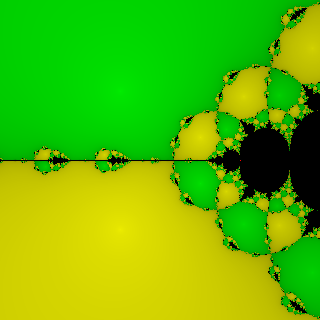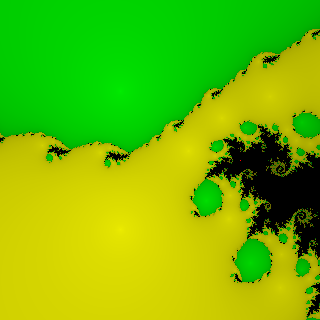$0.5$ $0.5+0.3i$$i$ $0.4+0.9i$

## Imitation

If you look again at the images in the above section, you may notice that some of the shapes – particularly the ones that occur when things are raised to real powers near 0.5 – are beginning to look interestingly like the shape of the Mandelbrot set. This suggested to me that there might be some sort of close relationship between these fractals and Mandelbrot/Julia sets.

So an obvious question to ask along these lines is, is there a function to which we could apply the Newton-Raphson formula $z \mapsto z - \frac{f(z)}{f'(z)}$ and end up with the Julia set iteration $z \mapsto z^2+c$?

Well, if you write down the equation $z - \frac{f(z)}{f'(z)} = z^2+c$, or equivalently $z - \frac{f}{df/dz} = z^2+c$, then it looks a lot like a differential equation. If only we were working in the positive reals instead of the complex numbers, we could solve it quite easily by separating variables into $df/f = -dz/(z^2-z+c)$, factorising the denominator of the RHS and turning it into partial fractions, and integrating to get $f = (z-a)^{1/(b-a)}(z-b)^{1/(a-b)}$, where $a$ and $b$ are the roots of the quadratic $z^2-z+c$. (Unless $c=\frac14$, which is a special case arising from $z^2-z+c$ being a perfect square and looks rather different; but I'll ignore that, because it's not a very interesting Julia set anyway.)

And it turns out that even in the complex numbers this answer works plausibly. The function $(z-a)^{1/(b-a)}(z-b)^{1/(a-b)}$ is of precisely the form discussed in the previous section: it's the product of linear terms raised to arbitrary powers. Hence we can conveniently do the Newton-Raphson iteration for this function by the method described above: compute $\frac1{(b-a)(z-a)} + \frac1{(a-b)(z-b)}$ and subtract its reciprocal from $z$. If you do that, remembering that by construction $a+b=1$ and $ab=c$, you will indeed find that a lot of algebraic mess cancels out and you end up with the iteration $z \mapsto z^2+c$.

So there is a family of functions to which the application of the Newton-Raphson formula yields a Julia set iteration, and moreover those functions aren't very far away from the ones we've already been considering here. To prove it works, here are a couple of plots of Julia sets, with their corresponding Newton-Raphson plot: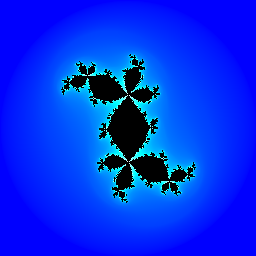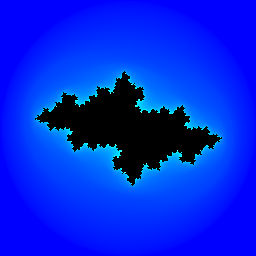Julia set for $0.28+0.528i$ Julia set for $-0.656+0.272i$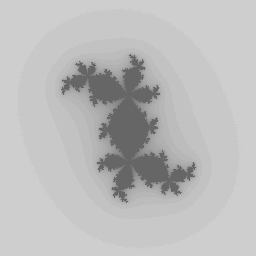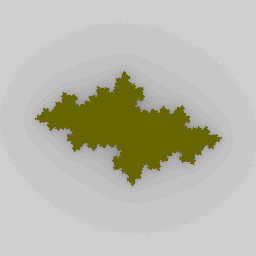Newton-Raphson fractal for $(z-(0.9994-0.5286i))^{-0.4722-0.4998i} \times$ $(z-(0.0006+0.5286i))^{0.4722+0.4998i}$ Newton-Raphson fractal for $(z-(1.4623-0.1413i))^{-0.5086-0.0747i} \times$ $(z-(-0.4623+0.1413i))^{0.5086+0.0747i}$

You will have noticed, of course, that the colouring is very different. The two types of fractal are using matching iteration formulae, but a Julia set plotter concentrates on how long it takes the iteration value to land outside a critical circle, whereas the Newton-Raphson plotter is actually waiting for the iterates to converge to a point, and is only incidentally observing what happens when they don't. So you wouldn't actually want to throw away your dedicated Julia-set plotting programs; but it's interesting, nonetheless, that something very like Julia sets are a special case of Newton-Raphson fractals.

## Dissection

Another noticeable thing about some of the above fractals is that some have much more fractal content than others. The original fractal at the top of this page with roots at $-1$, $+1$, $-i$, $+i$ has four big regions of flat colour meeting at the origin, and the self-similar nature of the fractal causes that quadruple contact point to be replicated in other parts of the plane. This gives rise to qualitatively more interesting fractal phenomena than the plot with seven roots in a line, in which all the large convergence regions are separated by boring curves which never meet, and the fractal blobs reflect this structure.

So I wondered, is there a way that we can predict how the convergence regions are going to be shaped, and thereby construct polynomials which have triple or quadruple meeting points exactly where we want them?

Yes, as it turns out, there is.

I observed above that raising a polynomial to a real power greater than 1 has the effect of causing the iteration to take smaller steps towards the root, which in turn causes convergence to be slower but surer and reduces the incidence of overshoot leading to fractal phenomena. As a result of this, the boundary lines between regions become clearer and smoother and simpler. This seemed like a good thing if we wanted to know the overall structure of the fractal; but merely clearer isn't good enough. I wanted clearest.

So I wondered what would happen "in the limit", as the iteration speed slowed down more and more. In other words, I was interested in what I'm going to describe as continuous Newton-Raphson, in which you start your "iteration" at an arbitrary point $z_0$ on the plane and then let $z$ evolve continuously according to the differential equation $\frac{dz}{dt} = -\frac{f(z)}{f'(z)}$.

As in the previous section, we can "solve" this equation by separating variables and naïvely integrating, trying hard to ignore the question of whether this is rigorous or even meaningful in the complex numbers. This time we end up with $\frac1f\frac{df}{dz}dz = -dt$, and then we can apply the integration-by-substitution formula to the LHS to give us $\frac{df}{f} = -dt$, which integrates to give us $f(z) = Ae^{-t}$ for some constant $A$. We can check by differentiating with respect to $t$ that this does indeed turn out to be a plausible answer to the equation even in the complex numbers: the chain rule tells us $\frac{d}{dt} f(z) = f'(z) \frac{dz}{dt}$, so we end up with $f'(z) \frac{dz}{dt} = -Ae^{-t} = -f(z)$ as required. And the constant $A$ is clearly equal to $f(z_0)$, the value of $f$ at the point where we started our integration.

"Very nice", I hear you protest, "but what does that mean?"

Well, since $t$ is a positive real, it means that whatever path $z$ follows from our starting point $z_0$ must have the property that at all times $f(z)$ is equal to $k f(z_0)$, where $k = Ae^{-t}$ is a real value which continuously and monotonically decreases from 1 toward 0. So we normally expect the limit of such an "iteration" to be a point at which $f(z)$ is actually equal to zero, i.e. a root of $f$.

But that's not the only thing that can happen. It's also possible to imagine that we might encounter a point along this path at which there isn't a clear direction we should head in: either there is no direction in which we can head from $z$ which will cause $f(z)$ to continue decreasing as a real multiple of its initial value, or perhaps there is more than one such direction.

How do we find such points? Well, for most points in the complex plane the differential equation we started with will usually give us a unique direction in which we can head to linearly decrease $f(z)$: we compute $f(z)/f'(z)$, and head in the direction pointed to by that complex number. This fails if $f(z)=0$, in which case there is no clear direction to head in; we expect that, of course, because if $f(z)=0$ then we've already reached a root. But it can also fail if $f'(z)=0$. So this suggests that the roots of the derivative of $f$ might be worth investigating.

So consider some root $r$ of $f'$, at which $f$ itself is non-zero. If we can find any continuous paths in the complex plane which start at $r$ and have $f$ increasing as a real multiple of $f(r)$, then any point on one of those paths will be a point at which starting a continuous Newton-Raphson process will cause it to head backwards along the same path and terminate at $r$ rather than at a root. In other words, we expect those paths to be precisely the boundaries between the convergence regions for the various roots (since that's the obvious set of points which we expect not to converge sensibly to a root); and moreover, on every such boundary we expect to find a root of $f'$ (because a continuous Newton-Raphson process started on any boundary has to end up somewhere).

That's a lot of maths to endure without a break for a pretty picture, and it's also a long and rather handwavey chain of reasoning to endure without some sort of reassurance that what I'm saying still makes sense. So, here I present a sample polynomial Newton-Raphson fractal, with the roots of the polynomial's derivative marked as black blobs. Observe that each dividing line between convergence regions has a blob somewhere on it, and that in particular the point where three regions (and three such lines) meet has a blob.

So now we know, at least in theory, how to find the lines dividing the different convergence regions. Now, what distinguishes a simple dividing line, separating only two regions, from a point at which three or more regions meet?

Let's consider our root $r$ of $f'$ again. We're now interested in how many directions we can head away from $r$ in, such that $f$ increases as a real multiple of $f(r)$. Equivalently, we're interested in directions we can head in such that $f(z)=(1+k)f(r)$ for some real positive $k$; in other words, we want small values $\epsilon$ such that $f(r+\epsilon)/f(r)-1$ is real and positive.

So now let's consider the Taylor series expansion for the function $f(r+\epsilon)/f(r)-1$. We have $$f(r+\epsilon) = f(r) + \epsilon f'(r) + \epsilon^2 \frac{f''(r)}{2!} + \epsilon^3 \frac{f'''(r)}{3!} + \cdots$$ and we know that $f'(r) = 0$ by construction, so this gives us $$\frac{f(r+\epsilon)}{f(r)}-1 = \epsilon^2 \frac{f''(r)}{2!f(r)} + \epsilon^3 \frac{f'''(r)}{3!f(r)} + \cdots$$

In the usual case, $f''(r)$ will be non-zero, so for small values of $\epsilon$, $f(r+\epsilon)/f(r)-1$ will be approximately equal to $K\epsilon^2$ for some (complex) constant $K$. This means that we expect to have two directions in which we can head in order to make $f(r+\epsilon)/f(r)-1$ real and positive: one direction corresponding to each square root of $1/K$. This fits with what we expect, because in the usual case a root of $f'$ gives rise to a region boundary extending away from it in two opposite directions.

But if $f''(r)$ is zero as well, then the $\epsilon^3$ term will now be the dominating one in the Taylor expansion; so for small values of $\epsilon$, $f(r+\epsilon)/f(r)-1$ will be approximately equal to $K\epsilon^3$ for some $K$. And now we expect to have three region boundaries coming out of our root $r$, one for each cube root of $1/K$. If the third derivative of $f$ is zero at $r$ too, then we can have four region boundaries, and so on.

And there it is: that's the result I've been working towards for this entire section. A multiple-region meeting point occurs when a root $r$ of $f'$ is also a root of $f''$, and the more higher derivatives are zero at $r$ the more regions meet there. For polynomials in particular, this translates into $r$ being a repeated root of $f'$, and the more repeated the merrier.

To demonstrate beyond reasonable doubt that this actually works, I will now construct from first principles a Newton-Raphson fractal containing two points at each of which five regions meet. For a five-way meeting point we need $f'$ to have a four-times-repeated root; so let's set $f'(z)=(z-1)^4(z+1)^4$, which has two repeated roots as desired. We "integrate" this in the naïve way (actually what we're doing is finding an anti-derivative of it, integration in the complex plane being a generally messy concept) to obtain the nasty-looking polynomial $(35z^9 - 180z^7 + 378z^5 - 420z^3 + 315z)/315$. We can discard the constant factor of $1/315$ since it makes no difference to the convergence, and this gives us $35z^9 - 180z^7 + 378z^5 - 420z^3 + 315z$. We find the roots of this (using Newton-Raphson for its more conventional purpose) and feed them into our fractal plotter, and get this picture:

And, exactly as we asked for, this has two five-way meeting points at locations $+1$ and $-1$. Bingo!

Of course, integration lets us add an arbitrary constant term without affecting the derivative of our result. Here's what we get if we add a constant term of $50+200i$ to the above polynomial:

The roots of the polynomial have moved around, and the picture is distorted, but the region meeting points are still exactly where we asked for them.

## Oscillation

Here's another curiosity. The following fractal is plotted using a pure polynomial $f$, placing the roots at $+i$, $-i$, $-2.3$ and $+2.3$:

The interesting feature of this picture is the black areas, which are regions in which Newton-Raphson failed to converge to a root within the program's limit of 256 iterations. At first sight one might assume that this is an artifact of having a finite iteration limit at all, but one would (it turns out) be wrong. Even if you crank up the iteration limit to a much larger number, those black areas stay black, because they represent genuine non-convergence.

What's actually happening here is that for this particular polynomial the Newton-Raphson method gives rise to period-2 cyclic behaviour. There's a pair of points $a$ and $b$, one in the middle of each of the two main black areas, which have the property that a single iteration of Newton-Raphson starting from $a$ takes you to $b$, and vice versa. But this is more than just a cycle between those two points: it's an attracting cycle. If you start from a point somewhere near $a$, an iteration of Newton-Raphson will take you to somewhere even nearer to $b$, from which another iteration will land you nearer still to $a$ again. Hence, there's a sizeable region around each point of the cycle which all converges to the cycle itself, and hence never settles down to a root of the polynomial.

This is interesting to me because it happens so rarely; I've plotted quite a lot of these fractals, including a lot of animated ones in which the points wander continuously around the plane, and this particular arrangement of roots is the only case I've found in which a polynomial gives rise to a non-converging area. (It's not the only actual polynomial: you can obviously rotate or translate the root positions to obtain other polynomials with the same behaviour, and you can also move the roots around by a small amount relative to each other before the black areas go away. But this general pattern – four roots in a diamond layout of roughly this shape – is the only pattern I know of that does this.)

On one level, it's reasonably easy to see "why" it happens. If you define $g(x) = x - f(x)/f'(x)$ to be the Newton-Raphson iteration for this polynomial, then find roots of the equation $g(g(x))=x$ (probably using a computer algebra package, since it's a pretty ugly mess) you will observe that it has roots which are not also roots of the similar first-order equation $g(x)=x$ (and hence of $f$). That proves that there are cycles at all; then, to prove there are attracting cycles, compute the derivative of $G(x)=g(g(x))$ at those roots and find the ones where it has modulus less than 1 (since for small $h$, $G(r+h)$ will be approximately $G(r)+G'(r)h$, and if $G(r) = r$ and $|G'(r)| < 1$ then this will be closer to $r$ than we started out).

But that doesn't really explain the phenomenon, or predict what other sorts of polynomial will give rise to cyclic behaviour of this type. Do there exist polynomials which exhibit period-$n$ cycles for all $n > 1$, for example? How often does this happen? How would one go about constructing polynomials with non-converging regions to order?

I don't currently know the answers to these questions, but I'd be interested to hear them if anyone else does.

## Participation

If you want to generate some of these fractal images yourself, you can download a program to generate them here: C source code and precompiled Windows executable. The program will produce PNG, Windows .BMP, or PPM files, and image processing software should be able to convert those to other formats if you prefer.

Just typing "newton" should give some help about what all the command-line options do. If you want a quick start, here's a selection of sample command lines you might like to try:

• newton -o simple.png -s 256x256 -x 2 -c 1,0,0:1,1,0:0,0.7,0:0,0.5,1 -- -1 +1 -i +i
This one is the cross-shaped plot I used at the top of this page, based on the polynomial $z^4-1$. To zoom in on one of the boundary lines as I did above, replace "-x 2" with "-x 0.4 -X 1.05 -Y 1.05".
• newton -o rainbow.png -s 320x256 -x 5 -c 1,0,0:1,0.7,0:1,1,0:0,1,0:0,0.7,1:0,0,1:0.5,0,1 -- -3 -2 -1 0 1 2 3
This one is the rainbow-coloured plot with seven roots strung out in a long line.
• newton -o powermap.png -s 256x256 -x 2 -c 1,0,0:1,1,0:0,1,0 -- 1/power -0.5-0.8660254i -0.5+0.8660254i
This command plots the various images from the large map shown above, with the red root raised to an arbitrary power. Replace power with the power you want; for example, replace it with 0.5 to get the Mandelbrot-like picture, and with 0.4+0.9i to get the disconnected yellow-and-green plot.
• newton -o holes.png -s 320x256 -y 2 -c 1,0,0:1,1,0:0,0.7,0:0,0.5,1 -- -2.3 +2.3 -i +i
This one is the plot with black non-converging areas.
• By default the program will use the cyclic shading behaviour with 16 shades of each colour. You can specify -C no to turn off cyclic shading (so the colours get uniformly darker as more iterations are needed), -B yes to turn on blurring of the iteration boundaries, and -f 32 or -f 64 if you want to increase the number of shades used.
• To raise the function to an overall power, use the -p option, for example -p 0.52. This will slow down convergence, so you will probably also need to increase the number of shades of colour (the -f option, as discussed above) in order to make the result look nice.
• To raise an individual root to a power other than 1, put a slash after that root followed by the power value. For example, an argument of 2+i/1-0.5i specifies a factor of $(x-(2+i))^{1-0.5i}$.
• If you want a larger version of an image, just change the picture size specified in the -s option.
• The program has a number of other options; just type newton on its own to list them.

Here are some pre-generated larger versions of the above images:

## Recognition

Newton-Raphson fractals are not a new idea of mine, although most other pages I've seen don't go so far into the maths. Here are a few such pages; you can probably find more by googling for "Newton-Raphson fractal".

• Alan Donovan was the person who introduced me to these fractals in the first place.
• Tom Womack also thought of animating them, although his page unfortunately doesn't include any actual movie files.
• Paul Bourke has some particularly well-chosen and pretty images.
• Bryan Krofchok has a more varied – and more colourful – gallery.
• MathWorld's page on the Newton-Raphson method itself mentions its fractal property, and has some small examples and further references.